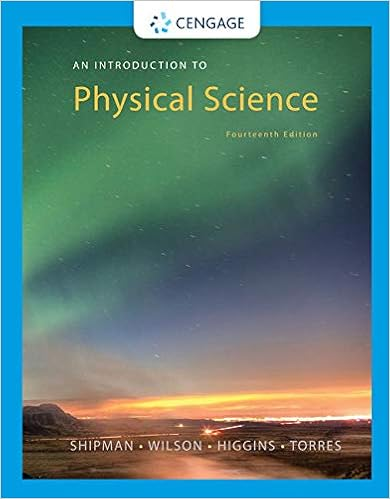# exam_final_17_for_18_soln.pdf - ECH 141Fluid Mechanics...

• Test Prep
• 8
• 88% (8) 7 out of 8 people found this document helpful

This preview shows page 1 - 3 out of 8 pages.

##### We have textbook solutions for you!
The document you are viewing contains questions related to this textbook.The document you are viewing contains questions related to this textbook.
Chapter 3 / Exercise 6
An Introduction to Physical Science
Shipman/WilsonExpert Verified
ECH 141—Fluid Mechanics Winter 2017 Final Exam 3/22/2017 (Two hours, closed book, no notes) 1. a) (10 pts.) Inertia is the dominant influence in a flow when the Reynolds number is (choose: high or low). When inertia is the dominant influence in a flow, a small region that forms near solid surfaces is called the _______________. b) (10 pts.) The _____________ equation is derived from the principle that mass is conserved. For an incompressible fluid, this equation may be stated as (choose one) i) the gradient of the velocity is zero or ii) the divergence of the velocity is zero.
b) (10 pts.) The continuityequation is derived from the principle that mass is conserved. For an incompressible fluid, this equation may be stated as (choose one) i) the gradient of the velocity is zero
. 2. (40 pts.) A Venturi meter is a gradual narrowing in a pipe that causes a pressure change. Measuring the pressure change, often with a manometer, allows one to infer the flow rate through the pipe. In the Venturi meter shown, fluid with density ρfat pressure p1flows through a pipe with cross-sectional area A1, enters the Venturi meter where the area for flow reduces gradually to A2, and then exits the Venturi meter and returns to the original diameter. A manometer containing a fluid with density ρmextends from a point at the entrance to the Venturi meter to its narrowest point. The flow is horizontal and all viscous effects may be neglected. Derive an expression that relates the volumetric flow rate Q through the pipe to H, A1, A2, ρfand ρm.
##### We have textbook solutions for you!
The document you are viewing contains questions related to this textbook.The document you are viewing contains questions related to this textbook.
Chapter 3 / Exercise 6
An Introduction to Physical Science
Shipman/WilsonExpert Verified
•••